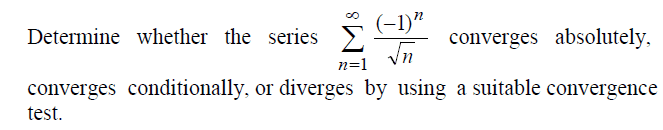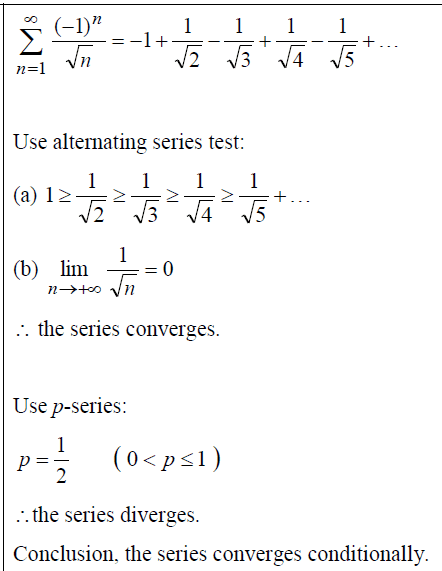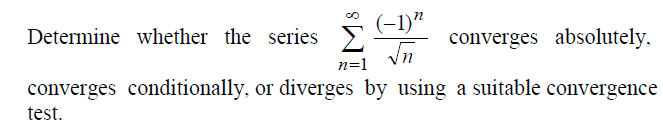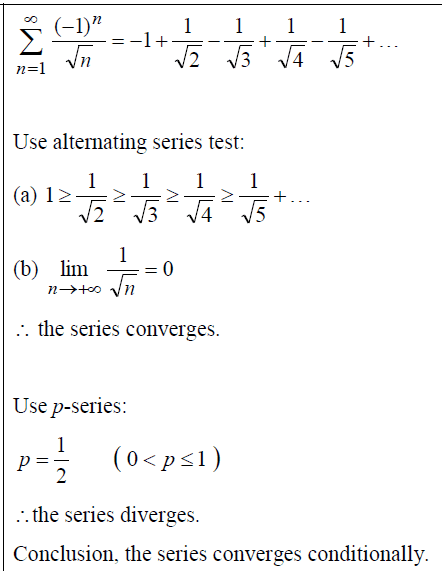# Convergence of series

• foo9008

## Homework StatementFrom the given ans , i knew that it's conditionally convergent (by using alternating test) i can understand the working to show that it's conditionally convergent . But , i also want to show it as not absolutely convergent ...

## The Attempt at a Solution

to prove that the series is not absolutely convergent , we would use ratio test , when the ratio is bigger than 1 , the series is said to be not absolutely convergent
however , i gt 1 (there's no conclusion whether the series is absolutely convergent or not )[/B]

https://en.wikipedia.org/wiki/Ratio_test

## Homework StatementFrom the given ans , i knew that it's conditionally convergent (by using alternating test) i can understand the working to show that it's conditionally convergent . But , i also want to show it as not absolutely convergent ...

## The Attempt at a Solution

to prove that the series is not absolutely convergent , we would use ratio test , when the ratio is bigger than 1 , the series is said to be not absolutely convergent
however , i gt 1 (there's no conclusion whether the series is absolutely convergent or not )[/B]

https://en.wikipedia.org/wiki/Ratio_test

By "wanna", do you mean "want to"?

Anyway, the image you pasted in your message already has a proof that the series is not absolutely convergent, so there is really nothing left to do.

The ratio test is a sufficient condition for convergence (and sometimes for divergence), but it says nothing useful when the limiting ratio equals 1. So, you need to use something else in that case.

BTW: I see that you claim "when the ratio is bigger than 1 , the series is said to be not absolutely convergent". A lot more than that is true: when the ratio is greater than 1 it is not even conditionally convergent (that is, the original series would be divergent).

Last edited:
The p-series is the test for absolute convergence.
To converge absolutely, the series:
##\sum_{n = 1}^ \infty \left| \frac{(-1)^n}{\sqrt{n}}\right|##
must converge.

If you insist on seeing the details, Wikipedia suggests using the integral test:
##\int_1^\infty \frac{1}{\sqrt{x}} dx\leq \sum_{n = 1}^ \infty \frac{1}{\sqrt{n}} \leq 1+ \int_1^\infty \frac{1}{\sqrt{x}} dx ##

•foo9008
By "wanna", do you mean "want to"?

Anyway, the image you pasted in your message already has a proof that the series is not absolutely convergent, so there is really nothing left to do.

The ratio test is a sufficient condition for convergence (and sometimes for divergence), but it says nothing useful when the limiting ratio equals 1. So, you need to use something else in that case.

BTW: I see that you claim "when the ratio is bigger than 1 , the series is said to be not absolutely convergent". A lot more than that is true: when the ratio is greater than 1 it is not even conditionally convergent (that is, the original series would be divergent).
do you mean to show that it's not absolutely convergent , we would use ratio test first , after found that it's not working for ratio test , then we used the p-series test to show that it's not absolutely convergent ?
P/s : the second picture is the solution given by the author

The p-series is the test for absolute convergence.
To converge absolutely, the series:
##\sum_{n = 1}^ \infty \left| \frac{(-1)^n}{\sqrt{n}}\right|##
must converge.

If you insist on seeing the details, Wikipedia suggests using the integral test:
##\int_1^\infty \frac{1}{\sqrt{x}} dx\leq \sum_{n = 1}^ \infty \frac{1}{\sqrt{n}} \leq 1+ \int_1^\infty \frac{1}{\sqrt{x}} dx ##
do you mean to show that it's not absolutely convergent , we would use ratio test first , after found that it's not working for ratio test , then we used the p-series test to show that it's not absolutely convergent ?

do you mean to show that it's not absolutely convergent , we would use ratio test first , after found that it's not working for ratio test , then we used the p-series test to show that it's not absolutely convergent ?

For the ##p##-series ##\sum_n 1/n^p## with ##p > 0##, the ratio test gives no useful information at all. In another thread we presented easy arguments (rather like the one given here in post #3) to demonstrate explicitly that the series diverges for ##0 < p \leq 1## and converges for ##p > 1##.

•foo9008
For the ##p##-series ##\sum_n 1/n^p## with ##p > 0##, the ratio test gives no useful information at all. In another thread we presented easy arguments (rather like the one given here in post #3) to demonstrate explicitly that the series diverges for ##0 < p \leq 1## and converges for ##p > 1##.
ok , what is the purpose of ratio test actually ?

ok , what is the purpose of ratio test actually ?

In some problems it is like the Holy Grail, but in this problem it is of no use at all. Whether or not it is useful depends very much on the individual problem details.

If you look back in this thread you will see that YOU were the person who first mentioned using the ratio test. So, what was YOUR thinking when you did that?

Last edited:
•foo9008
In some problems it is like the Holy Grail, but in this problem it is of no use at all. Whether or not it is useful depends very much on the individual problem details.

If you look back in this thread you will see that YOU were the person who first mentioned using the ratio test. So, what was YOUR thinking when you did that?
i was told to use the ratio test to test absolute convergence in my book ... is it ( the author) wrong ?

#### Attachments

In the book examples, the ratio test for absolute convergence is appropriate. As you have figured out, this problem falls under that category where no useful information can be gained from the ratio test.
If you were given this problem and explicitly told to use the ratio test, then I would stop at "no conclusion about convergence can be drawn from this test."
If you were told to determine if the series converges, as in this problem "by using a suitable convergence test," and the tests used had been introduced already, then I would expect you to eventually come to the conclusions given in the solution you posted.

i was told to use the ratio test to test absolute convergence in my book ... is it ( the author) wrong ?

I think you are mis-interpreting what the book says. It does say "Example 11: Use the ratio test for absolute convergence to detemine if the series converges". This is not a statement saying you should always use the ratio test; it is really saying "since this section is about the ratio test, let's use that test to check for the convergence of a couple of example series"

•foo9008
I think you are mis-interpreting what the book says. It does say "Example 11: Use the ratio test for absolute convergence to detemine if the series converges". This is not a statement saying you should always use the ratio test; it is really saying "since this section is about the ratio test, let's use that test to check for the convergence of a couple of example series"
so , to prove the series if it is absolutely convergent , there are many ways other than ratio test ?> since the ratio test stopped at no conclusion can be drawn from the test for the problem i posted in # post 1 , so we would use another method to prove it is not absolutely convergent ?

To prove that a series is (not) absolutely convergent, you simply need to show that the sum of the absolute values is (not) convergent. You may use any test or method to show that. As recommended above in post #3, the integral test works very well for the p-series.
##\int_1^\infty \frac{1}{\sqrt{x}} dx = \left. 2\sqrt{x} \right|_1^\infty. ##

so , to prove the series if it is absolutely convergent , there are many ways other than ratio test ?> since the ratio test stopped at no conclusion can be drawn from the test for the problem i posted in # post 1 , so we would use another method to prove it is not absolutely convergent ?

I believe I have already said that, twice.

Final word: it is common to start with the ratio test, because it is just about the simplest, most straightforward absolute-convergence test, and trying it may pay off. When it works you are done. When it does not work then, of course, you are forced to use some other method.

When I say "pay off" I mean that if the limiting ratio ##r## satisfies ##r < 1## you have convergence, and if ##r > 1## you have divergence. If ##r = 1## the test is inconclusive.

•foo9008